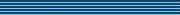| home      | people     | research     | publications    | seminars    | events     | contactCommunication Networks   | Systems Biology   | Hybrid Systems    | Machine Learning   | Dynamics & Interaction### Schools Mathematics Grand Challenge

Week four's Puzzles

Problem 10:

The solution was:

There are only 36 different amounts we could have shifted by, but in the code give, we can see the letter "I" alot. Since we know that "E" was originally the most common letter, which means that we have shifted by 6. So, the code looks like this:

```ABCDEFGHIJKLMNOPQRSTUVWXYZ0123456789
EFGHIJKLMNOPQRSTUVWXYZ0123456789ABCD
```

When we decode the message we get:

A BEEKEEPER KEEPS TWICE AS MANY BEES AS HE DOES SHEEP. HE KEEPS 625 SHEEP. HOW MANY BEES DOES THE BEEKEEPER KEEP

So the answer is 2*625 = 1250.

Problem 11:

The solution was:

Depending on your age and year in school, there will be different answers to this question. Suppose that I am 16 and that I am in third year in school. We want a number that when divided by 131 gives a remainder of 3 and when divided by 16 gives remainder 7.

Let's call the number we want n. We know that when we divide n by 131 we get a remainder of 3, so n = 131*m + 3, where m is a whole number. Now, we know that when we divide this number by 16 we get remainder 7. If we divide each part of n by 16 we get:
n = 131*m + 3 = 8*16*m + 3*m + 3

so the only bits that can give a remainder are 3*m + 3, as the other bit is divisible by 16. So we want 3*m+3 to give remainder 7 when divided by 7. Let's try a few numbers.

```m    3*m    3*m+3     remainder
-------------------------------
1      3      6          6
2      6      9          9
3      9     12         12
4     12     15         15
5     15     18          2
6     18     21          5
7     21     24          8
8     24     27         11
9     27     30         14
10    30     33          1
11    33     39          4
12    39     42          7
```

So, we can use m = 12 to get remainder 7.

So, for a 16 year old third year, one possible answer is 131*12+3 = 1575. There are other answer numbers which also work - you can get these by adding 131*16 to this number.

For other combinations of year and age, you can look up an answer in the following table. If you add 131*A you will also get another possible answer.

```                       Year
|  1       2       3       4       5       6
Age  |----------------------------------------------
12   |  787     919     1051    1183    1315    1447
13   |  787     657     527     397     267     137
14   |  525     133     1575    1183    791     399
15   |  787     1312    1837    397     922     1447
16   |  263     919     1575    135     791     1447
17   |  1180    2098    789     1707    398     1316
18   |  1573    133     1051    1969    529     1447
```

You could also solve this problem with the Chinese Remainder Theorem, if you know it!

Problem 12:

The solution was:

Substitution Ciphers are much harder to break than Caesar Ciphers, because there are many more of them. For 26 letters there are 403291461126605635584000000 different substitution ciphers. We gave each of you a different substitution cipher, but the same message. For me, the message was:

```P fext ef xwt ubtpvyfm eo deatg utoebt pgvyfm xwt cstgxyef.

Xwt gxsaz eo rbyxyfm pfa ubtpvyfm p gtdbtx deat yg dphhta dbzqxehemz
pfa yg eoxtf p jpxwtjpxydph xpgv. Xeapz deatg pbt phh jebt dejqhtk
xwpf nsgx grpqqyfm pbesfa htxxtbg. Gejt deatg pbt wpba xe ubtpv
utdpsgt opdxebygyfm p fsjutb ryxw p wsfabta aymyxg yg wpba. Yf xwt
Yfxtbftx, rwtf qteqht usz xwyfmg obej xwt rtu, xwtyb atxpyhg pbt
qbextdxta uz gejt opdxebygpxyefg!

Yf xwyg rttv'g qbeuhtj rt rpfx xwt pfgrtb xe eft cstgxyef: Wer jpfz
xyjtg aetg xwbtt ayiyat yfxe gyk wsfabta pfa xwybxz xwbtt?
```

First, we count which are the most common letters. In this paragraph we see:

```T       60 times
X       44 times
P       35 times
E       33 times
Y       32 times
F       31 times
G       30 times
```

So we know T<->E, X<->T, P<->A, E<->O, Y<->I, F<->N, G<->S. If we swap these letters in, we get:

```A NOTE ON T_E __EA_IN_ O_ _O_ES _E_O_E AS_IN_ T_E __ESTION.

T_E ST___ O_ __ITIN_ AN_ __EA_IN_ A SE__ET _O_E IS _A__E_ ____TO_O__
AN_ IS O_TEN A _AT_E_ATI_A_ TAS_. TO_A_ _O_ES A_E A__ _O_E _O___E_
T_AN __ST S_A__IN_ A_O_N_ _ETTE_S. SO_E _O_ES A_E _A__ TO __EA_
_E_A_SE _A_TO_ISIN_ A N___E_ _IT_ A __N__E_ _I_ITS IS _A__. IN T_E
INTE_NET, __EN _EO__E ___ T_IN_S __O_ T_E _E_, T_EI_ _ETAI_S A_E
__OTE_TE_ __ SO_E _A_TO_ISATIONS!

IN T_IS _EE_'S __O__E_ _E _ANT T_E ANS_E_ TO ONE __ESTION: _O_ _AN_
TI_ES _OES T__EE _I_I_E INTO SI_ __N__E_ AN_ T_I_T_ T__EE?
```

where "_" is the letters we don't yet know.

Now we just have to spot words. For example "T_E" is probably "THE", so we know W<->H. "__ESTION" is probably "QUESTION", so C<->Q and S<->U. "AN_" is probably "AND" and "O_TEN" is probably "OFTEN", so A<->D and O<->F.

Eventually, we can decode the whole paragraph to get:

```A note on the breaking of codes before asking the question.

The study of writing and breaking a secret code is called cryptology
and is often a mathematical task. Today codes are all more complex
than just swapping around letters. Some codes are hard to break
because factorising a number with a hundred digits is hard. In the
Internet, when people buy things from the web, their details are
protected by some factorisations!

In this week's problem we want the answer to one question: How many
times does three divide into six hundred and thirty three?
```

so the answer is 633/3 = 211.